Novel Games - Mastering All the Games in Human History
search Play Games Forums License Our Games
Name
0 / 2455
0
99

Settings Log Out

English

# A Simple Set of Formulas for Magic Cube

by Novel Games
2008-01-10 15:50:51
#1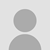There are many ways to solve Rubik's Cube, but they all involve the use of formulas to move the blocks. Some solutions require the use of more than 50 formulas which is rather difficult to learn. The following is a set of 3 formulas invented by late Mr. Ngai Shing Lee, and this set of formulas is easy to learn and remember. Note that a lot of moves is needed and you cannot solve the puzzle extremely quickly, but eventually you will be able to solve it.

The basic concept is to solve the corners first and then the edges. This is based on a simple observation that moving the middle rows alone will not disrupt the corners.

The basic procedure is as follows:
1. Move the four corners of one face to their correct positions and correct orientations (you should not need any formulas for this)
2. Use formula A to move the remaining four corners to their correct positions but not necessarily in correct orientations
3. Use formula B to rotate the remaining corner blocks to their correct orientations. Now all eight corners should be correct
4. Use formula C to move the edges to their correct positions
All three formulas will move the blocks in the bottom face while keeping the top face intact. The basic step in the formulas is to "remove a block from the top face and then put it back".

Now start with formula A, which swaps the positions of 3 corner blocks:(For easy demonstration, the result shown above is starting with a solved cube)

Formula A goes as follows: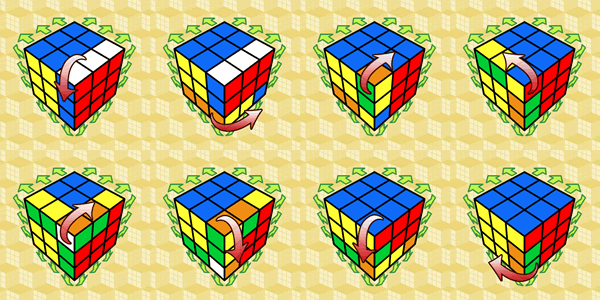Note that the first 3 steps involve the removal of the lower right corner of the top face, and the remaining steps are to put it back. You can also apply a variation of this formula which removes and puts back the lower left corner of the top face to achieve a similar effect for the top left, bottom left and bottom right blocks on the bottom face.

After you have finished moving the corner blocks of the bottom face to their correct positions (but not necessarily in correct orientations), you can apply formula B to rotate the blocks counterclockwise: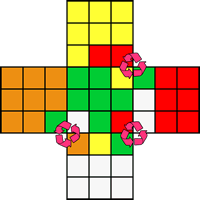Formula B goes as follows: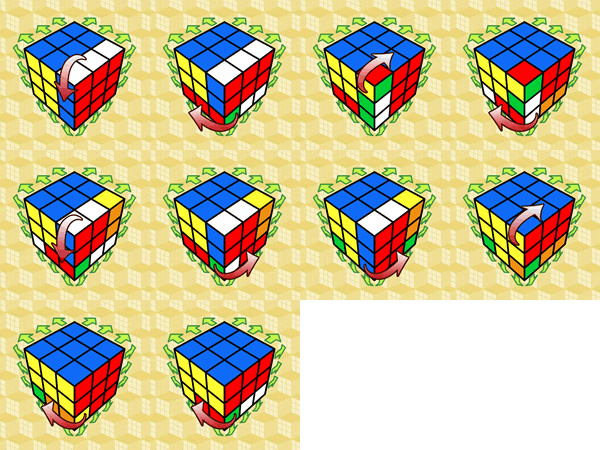Note that the first 3 steps involve the removal of the lower right corner of the top face, and the remaining steps are to put it back. If you apply a variation of this formula, the top left, bottom left and bottom right blocks in the bottom face will be rotated clockwise.

After you have finished all the 8 corners, you should deal with the edges using formula C, which swaps the positions of 3 blocks on the edge in a counterclockwise direction: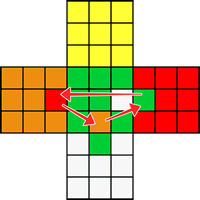Formula C goes as follows:Note that the first 3 steps involve the removal of the lower middle block, and the remaining steps are to put it back. You can also apply a variation of this formula to swap the positions of the blocks in a clockwise direction by swapping the left and right rotations as shown above.

When using formula C, sometimes you will face a situation that the incorrect blocks are not on the same face, and in this case you will need to make extra moves to move the incorrect blocks to the same face before applying formula C.
by Novel Games
2008-01-10 15:50:51
Like